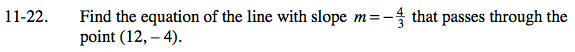Home > AC > Chapter 11 > Lesson 11.1.2 > Problem11-22

11-22.

Find the equation of the line with slope m =that passes through the point (12, −4). Homework Help ✎Substitute the slope and the coordinates into the Slope-Intercept form of a line to solve for b.
Then, rewrite the equation with only the values for m and b substituted in.

See problem 10-85 for help finding the equation of a line.

$y=-\frac{4}{3}x+12$